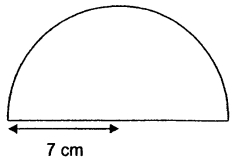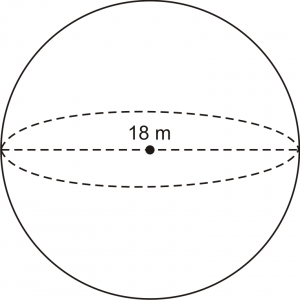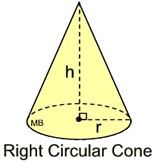## How to Calculate and Solve for the Centroid or Centre of Gravity of a HemisphereThe image above is a hemisphere with a radius of 5.
To compute the centroid or centre of gravity of a hemisphere. You need one essential parameter and this parameter is the radius of the hemisphere (r).

The formula for calculating the centroid or centre of gravity of a hemisphere is:

C.G. = 3r / 8

Where
r = Radius of the hemisphere

As always let us try and solve an example:
Find the centroid or centre of gravity of a hemisphere where the radius is 5 cm.

From the formula this implies that:
r = Radius of the hemisphere = 5

C.G. = 3(5) / 8
C.G. = 15 / 8
C.G. = 1.875

Therefore, the centroid or centre of gravity of the hemisphere is 1.875.

Nickzom Calculator – The Calculator Encyclopedia is capable of calculating the centre of gravity of a hemisphere at a distance from its base measured along the vertical radius.

To get the answer and workings of the center of gravity or centroid of a hemisphere using the Nickzom Calculator – The Calculator Encyclopedia. First, you need to obtain the app.

You can get this app via any of these means:

## Nickzom Calculator Calculates the Centroid or Centre of Gravity of a Semicircle | Statics (Mechanics)The image above is a semicircle with a radius of 7 cm.
To compute the centroid or centre of gravity of a semicircle, you need one essential parameter and this parameter is the radius of the semicircle.

The formula for calculating the centroid or centre of gravity of a semicircle is:

C.G.= 4r /

Where:
r = Radius of the Semicircle
π = Mathematical Constant = 3.142 (approximately)

Let’s solve an example:
Find the centroid or centre of gravity of a semicircle where the radius is 7 cm.

From the example above,
r = radius of the semicircle = 7

C.G. = 4(7) /
C.G. = 28 / 9.4247
C.G. = 2.97

Therefore, the centroid or centre of gravity of the semicircle is 2.97.

Nickzom Calculator – The Calculator Encyclopedia is capable of calculating the centre of gravity of a semicircle at a distance from its base measured along the vertical radius.

To get the answer and workings of the center of gravity or centroid of a semicircle using the Nickzom Calculator – The Calculator Encyclopedia. First, you need to obtain the app.

## How to Calculate and Solve for the Centroid or Centre of Gravity of a SphereThe image above is a sphere and 18 m is the diameter of the sphere. In the computing of the centroid or centre of gravity of a sphere there is only one essential parameter which is the diameter of the sphere.

The formula for calculating the centroid or centre of gravity of a sphere is:

C.G. = d / 2

Where:

d is the diameter of the sphere

As always, let’s take an example:

Let’s solve an example
Find the centroid or centre of gravity of a sphere where the diameter is 18 m.

C.G. = 18 / 2
C.G. = 9

Therefore, the centroid or centre of gravity of the sphere is 9.

Nickzom Calculator – The Calculator Encyclopedia is capable of calculating the centre of gravity of a sphere at a distance from every point.

To get the answer and workings of the center of gravity or centroid of a sphere using the Nickzom Calculator – The Calculator Encyclopedia. First, you need to obtain the app.

You can get this app via any of these means:

## How to Calculate and Solve the Centre of Gravity of a Right Circular ConeThis image above is a display of what a right circular cone looks like. There is only one essential parameter for calculating the centroid or centre of gravity of a right circular cone. This parameter is the height of the cone (h).

The formula for calculating the the centroid or centre of gravity of a right circular cone is:

C.G. = h / 4

As always let’s solve an example.

Find the centroid or centre of gravity of a right circular cone where the height of the cone is 12cm.
This implies that:

h = height of the cone = 12

C.G. = 12 / 4
C.G. = 3

Therefore, the centroid or centre of gravity of the right circular cone is 3.

Nickzom Calculator – The Calculator Encyclopedia is capable of calculating the centre of gravity of a right circular cone at a distance from its base measured along the vertical axis.

To get the answer and workings to center of gravity or centroid of a right circular cone using the Nickzom Calculator – The Calculator Encyclopedia. First, you need to obtain the app.

You can get this app via any of these means:

## How to Calculate the Centre of Gravity of a Circular Sector in Statics | Mechanics

It is very possible to compute the centroid or centre of gravity of a circular sector. There are two highly important parameters one needs to know to compute the centre of gravity of a circular sector. These parameters are:

• Radius of the Sector (r)
• Semi Vertical Angle (α)

The formula for computing the centre of gravity of a circular sector is:

C.G. = 2rsinα /

Now, let’s take an example.
Let’s find the centroid or the centre of gravity of a circular sector that has a radius of 4m and a semi vertical angle of 30°.
This implies that:

r = Radius of the Sector = 4
α = Semi Vertical Angle = 30

Entering this values into the formula we have:

C.G. = 2(4)sin30° / 3(30)
C.G. = 8 . sin30° / 90
C.G. = 8 . (0.5) / 90
C.G. = 4 / 90
C.G. = 0.0444

Therefore, the centroid or centre of gravity of the circular sector is 0.0444.

Nickzom Calculator – The Calculator Encyclopedia is capable of calculating the centre of gravity of a circular sector making a semi vertical angle α at a distance from the centre of the sector measured along the central axis.

To get the answer and workings to center of gravity or centroid of a circular sector. First, you need to obtain the Nickzom Calculator – The Calculator Encyclopedia app.

## Nickzom Calculator Calculates the Center of Gravity of a Trapezium in Statics Mechanics

According to Quora,

The center of gravity of a trapezoid can be estimated by dividing the trapezoid in two triangles.

Nickzom Calculator calculates the centre of gravity of a trapezium with parallel sides a and b at a distance measured from side b.

The formula for calculating this center of gravity is:

C.G. = h (b + 2a) / 3 (b + a)

Where, a and b are the length of the parallel sides of the trapezium (b being the base length and a being the top length) whereas h is the height of the trapezium.

Let’s take for Example: Find the center of gravity or centroid of a trapezium where a is 4, b is 8 and h is 2.

This implies:

a = 4
b = 8
h = 2

C.G. = 2 (8 + 2(4)) / 3 (8 + 4)
C.G. = 2 (8 + 8) / 3 (12)
C.G. = 2 (16) / 36
C.G. = 32 / 36
C.G. = 0.89

Therefore, the center of gravity or centroid of the trapezium is 0.89.

To get the answer and workings to center of gravity or centroid of a trapezium. First, you need to obtain the Nickzom Calculator – The Calculator Encyclopedia app.